# Chapter 1 Step into R programming–the iris flower dataset

Learning objectives:

• Install R and Rstudio.
• Data frames and vectors.
• The absolute basics way to analyze data with R: import data, check data types, attach, and then plot and analyze using column names.

## 1.1 Getting started

1. Install R from www.R-project.org. Choose the cloud server or any mirror site.

2. Install RStudio Desktop from www.RStudio.com. RStudio uses R in the background but provides a more user-friendly interface. R commands can be typed directly into the “Console” window. Or you can enter them in the “R Script” window and click the “Run” button. If you have an older version of R or Rstudio, we recommend upgrading to the latest version.

3. Alternatively, you can use R and Rstudio through a web browser via Rstudio Cloud.. Sign up to create a free account, or just log-in with your Google account. Click the “New Project” button to get started.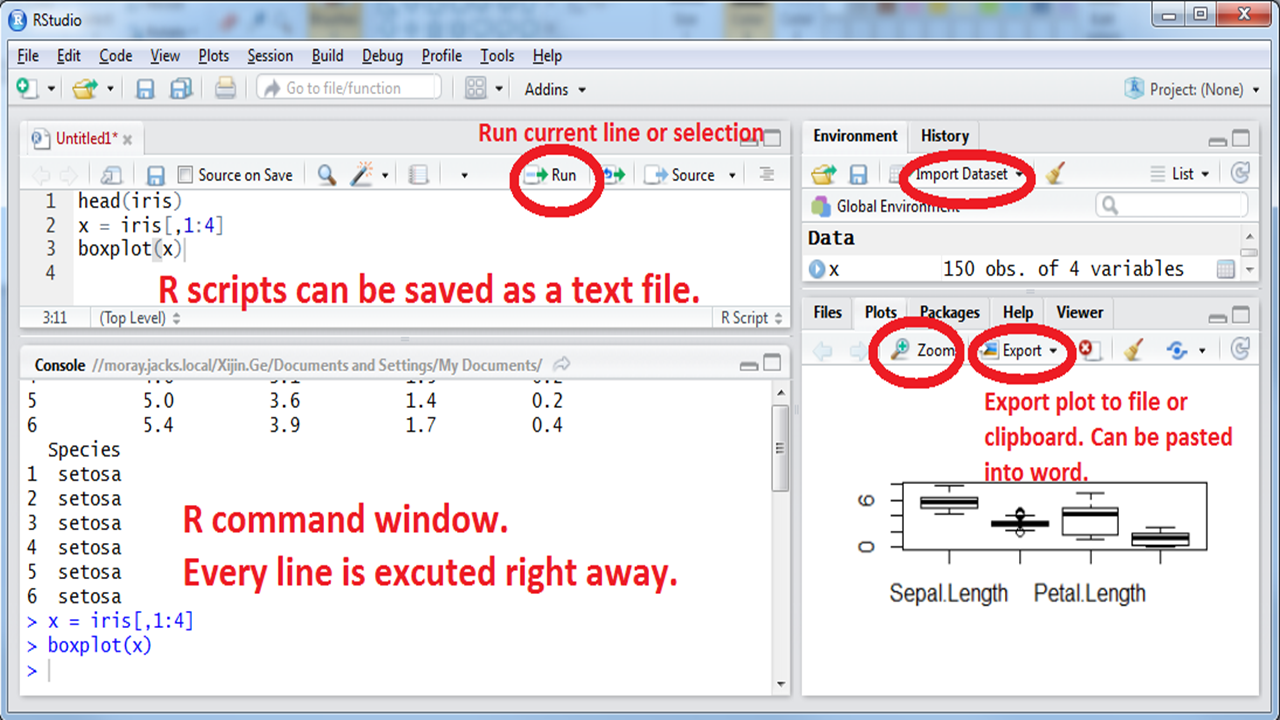As a quick sample session, let’s try all of these commands below in the Console window. R is case-sensitive; “Species” and “species” are two different variables. If you understand all of these, you probably do not need this book; consider more advanced books like R for Data Science. If it takes a few months to type these 243 characters, try www.RapidTyping.com first.

head(iris)
str(iris)
summary(iris)
x <- iris[, 1:4]
boxplot(x)
plot(x[, 3:4])
abline( lm(x[, 4] ~ x[, 3]) )
pairs(x)
stars(x)

PL <- x$Petal.Length PL barplot(PL) hist(PL) Species <- iris$Species
Species
pie( table(Species) )
boxplot(PL ~ Species )
summary( aov(PL ~ Species) )Animated GIF for screen shot.

## 1.2 Data frames contain rows and columns: the Iris flower dataset

In 1936, Edgar Anderson collected data to quantify the geographic variations of Iris flowers. The data set consists of 50 samples from each of the three sub-species ( Iris setosa, Iris virginica, and Iris versicolor). Four features were measured in centimeters (cm): the lengths and the widths of both sepals and petals. This is one of many built-in datasets in R. Download this dataset from GitHub, and open it in Excel. Have a quick look at the data, think about what distinguishes the three species. If we have a flower with sepals of 6.5cm long and 3.0cm wide, petals of 6.2cm long, and 2.2cm wide, which species does it most likely belong to? Think (!) for a few minutes while eyeballing the data. Write down your guessed species. Getting familiar with this dataset is vital for this and next chapters.Figure 1.1: Iris flower. Photo from Wikipedia.

If you are using Rstudio Cloud through a web browser, you are using a computer on the cloud, which cannot directly access your local files. Therefore you need to first upload the data file to the cloud using the Upload button in the Files tab in the lower right panel.

The data file can be imported into R by selecting Import Dataset in the File menu, and then choose “From Text(base)…”. As the default settings work well in this case, we just need to click Import in the pop-up window. That’s it! There is no need to spend half of a semester to learn how to import data, like some people used to complain when learning SAS. If you have trouble importing this data file, you can skip this step as this data set is included in R.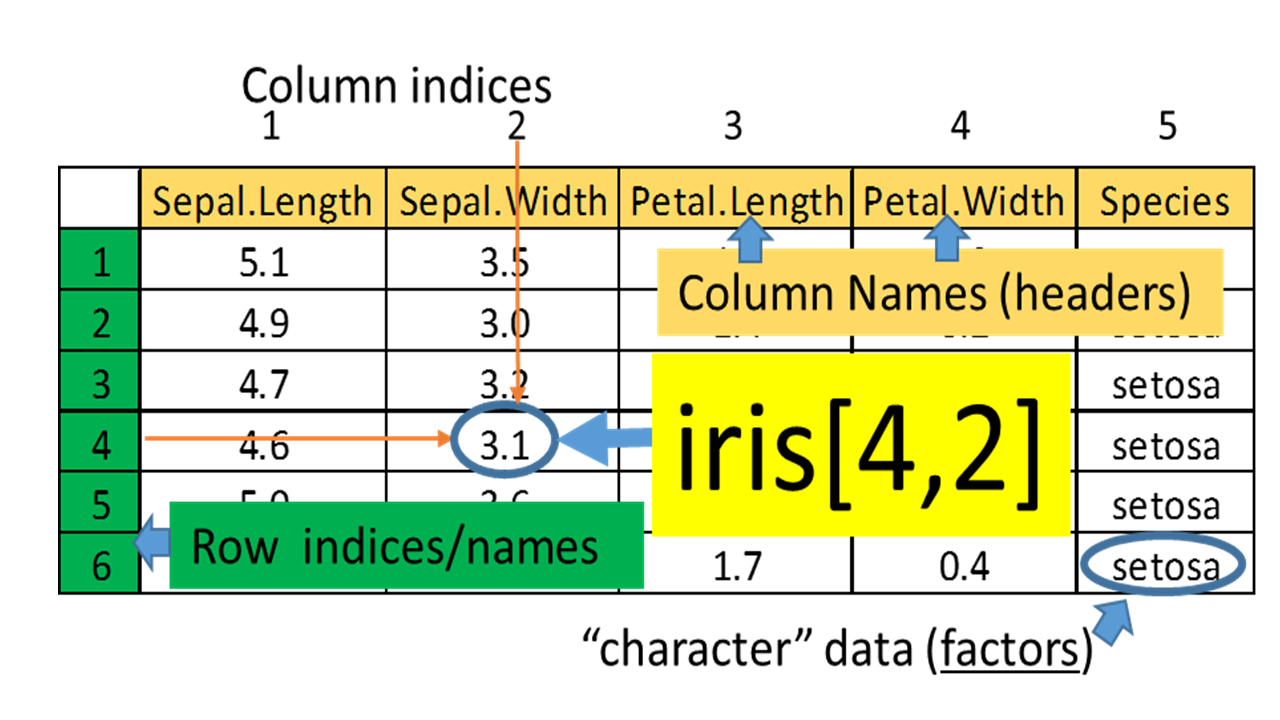Figure 1.2: Example of a data frame.

To answer these questions, let us visualize and analyze the data with R. Type these commands without the comments marked by “#.”

View(iris) # show as a spreadsheet
head(iris)  # first few rows
##   Sepal.Length Sepal.Width Petal.Length Petal.Width Species
## 1          5.1         3.5          1.4         0.2  setosa
## 2          4.9         3.0          1.4         0.2  setosa
## 3          4.7         3.2          1.3         0.2  setosa
## 4          4.6         3.1          1.5         0.2  setosa
## 5          5.0         3.6          1.4         0.2  setosa
## 6          5.4         3.9          1.7         0.4  setosa
str(iris)   # show the structure
## 'data.frame':    150 obs. of  5 variables:
##  $Sepal.Length: num 5.1 4.9 4.7 4.6 5 5.4 4.6 5 4.4 4.9 ... ##$ Sepal.Width : num  3.5 3 3.2 3.1 3.6 3.9 3.4 3.4 2.9 3.1 ...
##  $Petal.Length: num 1.4 1.4 1.3 1.5 1.4 1.7 1.4 1.5 1.4 1.5 ... ##$ Petal.Width : num  0.2 0.2 0.2 0.2 0.2 0.4 0.3 0.2 0.2 0.1 ...
##  $Species : Factor w/ 3 levels "setosa","versicolor",..: 1 1 1 1 1 1 1 1 1 1 ... The imported data is stored in a data frame called iris, which contains information on 5 variables for 150 observations (flowers). While the first 4 variables/columns contain numeric values, the 5th column contains characters indicating which sub-species a sample belongs to. This is an important distinction, as they are treated differently in R. Sometimes we need to overwrite data types guessed by R, which can be done during the data importing process. If you are using the built-in iris data, the Species column is a factor with three levels. summary(iris) # summary statistics ## Sepal.Length Sepal.Width Petal.Length Petal.Width ## Min. :4.300 Min. :2.000 Min. :1.000 Min. :0.100 ## 1st Qu.:5.100 1st Qu.:2.800 1st Qu.:1.600 1st Qu.:0.300 ## Median :5.800 Median :3.000 Median :4.350 Median :1.300 ## Mean :5.843 Mean :3.057 Mean :3.758 Mean :1.199 ## 3rd Qu.:6.400 3rd Qu.:3.300 3rd Qu.:5.100 3rd Qu.:1.800 ## Max. :7.900 Max. :4.400 Max. :6.900 Max. :2.500 ## Species ## setosa :50 ## versicolor:50 ## virginica :50 ## ## ##  The summary function provides us with information on the distribution of each variables. There are 50 observations for each of the three sub-species. Individual values in a data frame can be accessed using row and column indices. iris[3, 4] # value in 3rd row, 4th column: 0.2. iris[3, ] # all of 3rd row iris[, 4] # all of column 4 iris[3, 1:4] # row 3, columns 1 to 4 To do the exercises, you need to create a Word document. Then copy-and-paste the R code and results to it. Save it as a PDF file. Submit both the Word and PDF files to the Dropbox. Exercise 1.1 Type data() in the R Console window. The pop-up window - R data sets - contain all built-in data sets in package ‘datasets.’ Choose a data set whose structure is data frame, then answer the following questions: 1. Display the first few rows of the data set. NOT all values in your data set. 2. Show the dimension of the data set. 3. Extract a subset which contains values in the 2nd through the 5th rows and the 1st through the 4th columns. If your data set contains fewer rows or columns, please choose another data set. You can replace the iris data set with any of the built-in data sets as long as its data structure is data frame. Make sure you check the structure of the data set by the class() function. Note: You will need to change the variable names and ranges of rows and columns.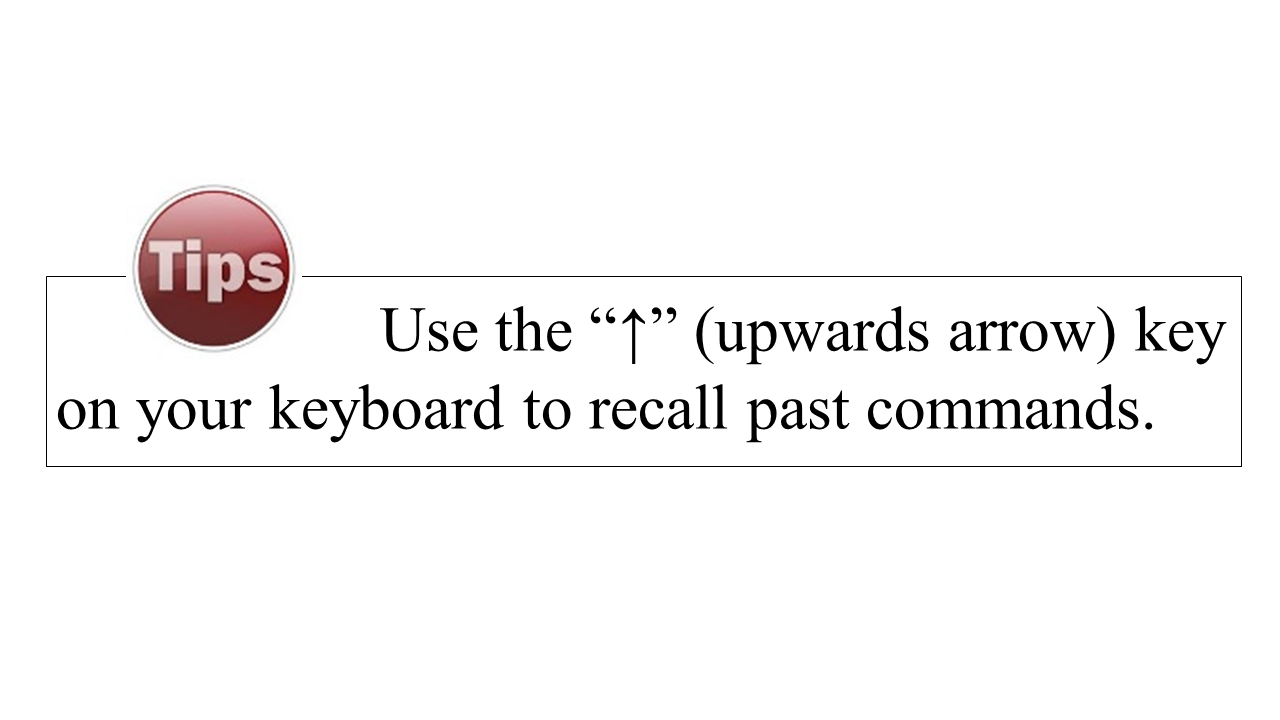colnames(iris) # Column names ##  "Sepal.Length" "Sepal.Width" "Petal.Length" "Petal.Width" "Species" Remember these column names, as we are going to use them in our analysis. Note that sepal length information is contained in the column named Sepal.Length. We can retrieve an entire column by using the data frame name followed by the column name. R is case-sensitive. “iris$petal.length” will not be recognized.

iris$Petal.Length # 150 petal lengths  This is the recommended, safer way of referring to columns. But if you are as lazy as me and do not want to type the data frame name repeatedly. This is a shortcut by attaching a data frame the columns can be accessed directly. One disadvantage is that it will cause issues if two datasets have the same variable names, or if the data is transformed or changed later on. We typically do not recommend doing it but here we want to make things simple. We should attach a dataset after we are sure that we will not making changes to it. attach(iris) # Forget this sneaky shortcut after chapter 3! Petal.Length # 150 petal lengths mean(Petal.Length) # mean( ) is a function that operates on Petal.Length ##  3.758 Exercise 1.2 1. Compute the mean of the sepal length in the data set iris. 2. Compute the mean of speed in the built-in data set cars. The best way to find and learn R functions is Google search. The R community is uniquely supportive. There are many example codes and answers to even the dumbest questions on sites like StackOverflow.com. Exercise 1.3 1. Google “R square root function” to find the R function, and compute the value of $$\sqrt{56.7}$$. 2. Use R to find the maximal value of mpg in the data set mtcars. ## 1.3 Analyzing one set of numbers First, let us retrieve the 150 numbers in the petal length column and store it in an vector called PL. PL <- iris$Petal.Length   

Some might complain that nothing happens after you type in this command. Although nothing is printed out, you have created a new vector called PL in the memory. You can find it in the top right panel in the Environment tab. The process of programming is all about creating and modifying data objects.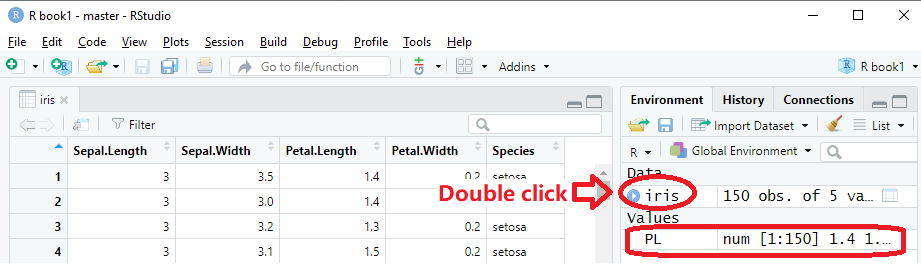A vector holds a series of numbers or characters. R made it very easy to do the same calculations (add, subtract, multiply,…) on all of them at once or collectively.

summary(PL)
##    Min. 1st Qu.  Median    Mean 3rd Qu.    Max.
##   1.000   1.600   4.350   3.758   5.100   6.900

This produces a lot of useful information about the distribution of petal length:

• The minimum is 1.0, and the maximum is 6.9.

• Average petal length is 3.758.

• The mid-point, or median, is 5.35, as about half of the numbers are smaller than 5.35. Why the median is different from the mean? What happens if there is a typo and one number is entered 340cm instead of 3.40cm?

• The 3rd quartile, or 75th percentile, is 5.1, as 75% of the flowers have petals shorter than 5.1. If a student’s GPA ranks 5th in a class of 25, he/she is at 80th percentile.

• The 1st quartile, or 25th percentile, is 1.6. Only 25% of the flowers have petals shorter than 1.6. These summary statistics are graphically represented as a boxplot in the Figure 1.3A. Boxplots are more useful when multiple sets of numbers are compared.

boxplot(PL)   # Figure 1.3A.
boxplot(iris[, 1:4])  # boxplot Figure 1.3B.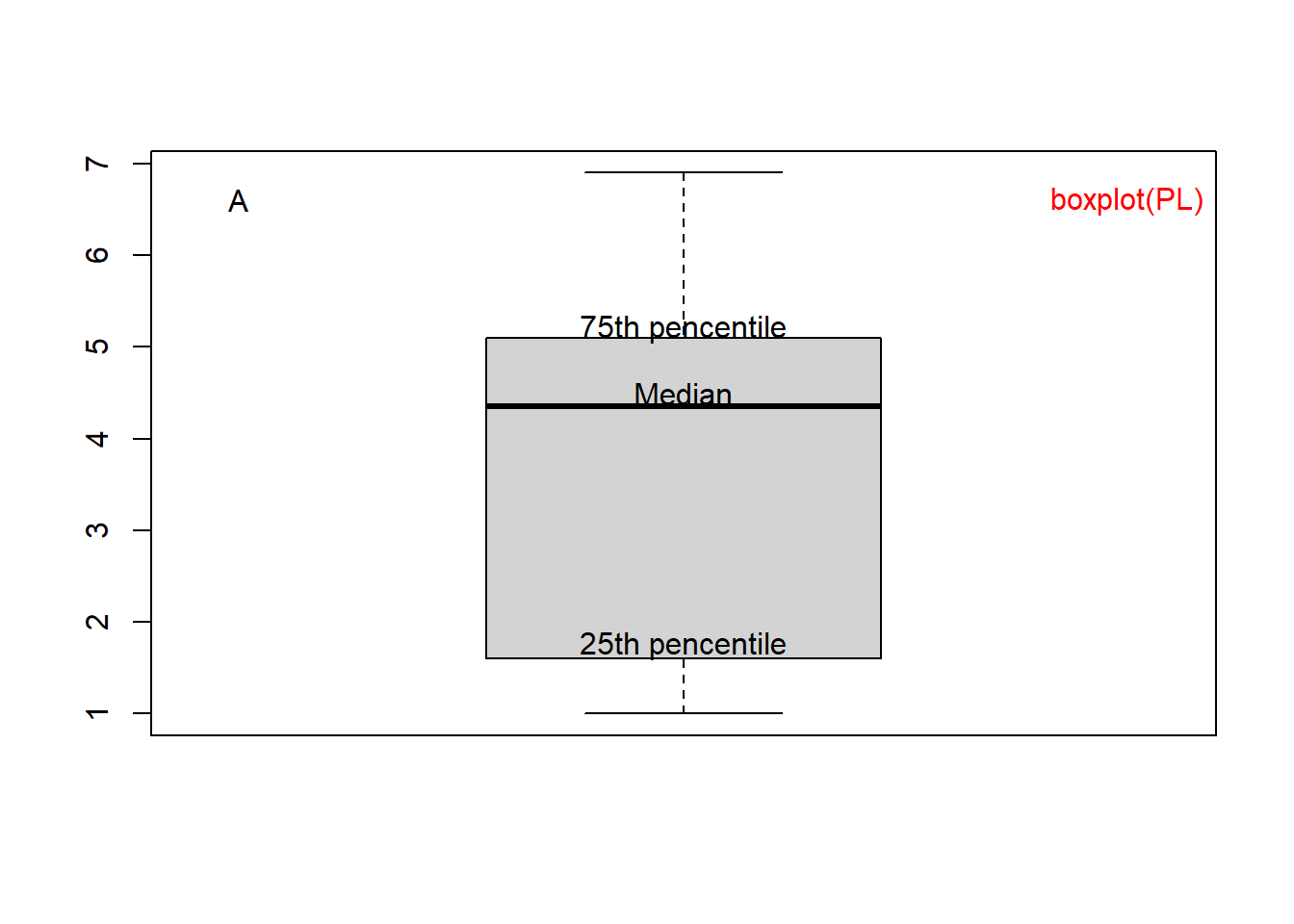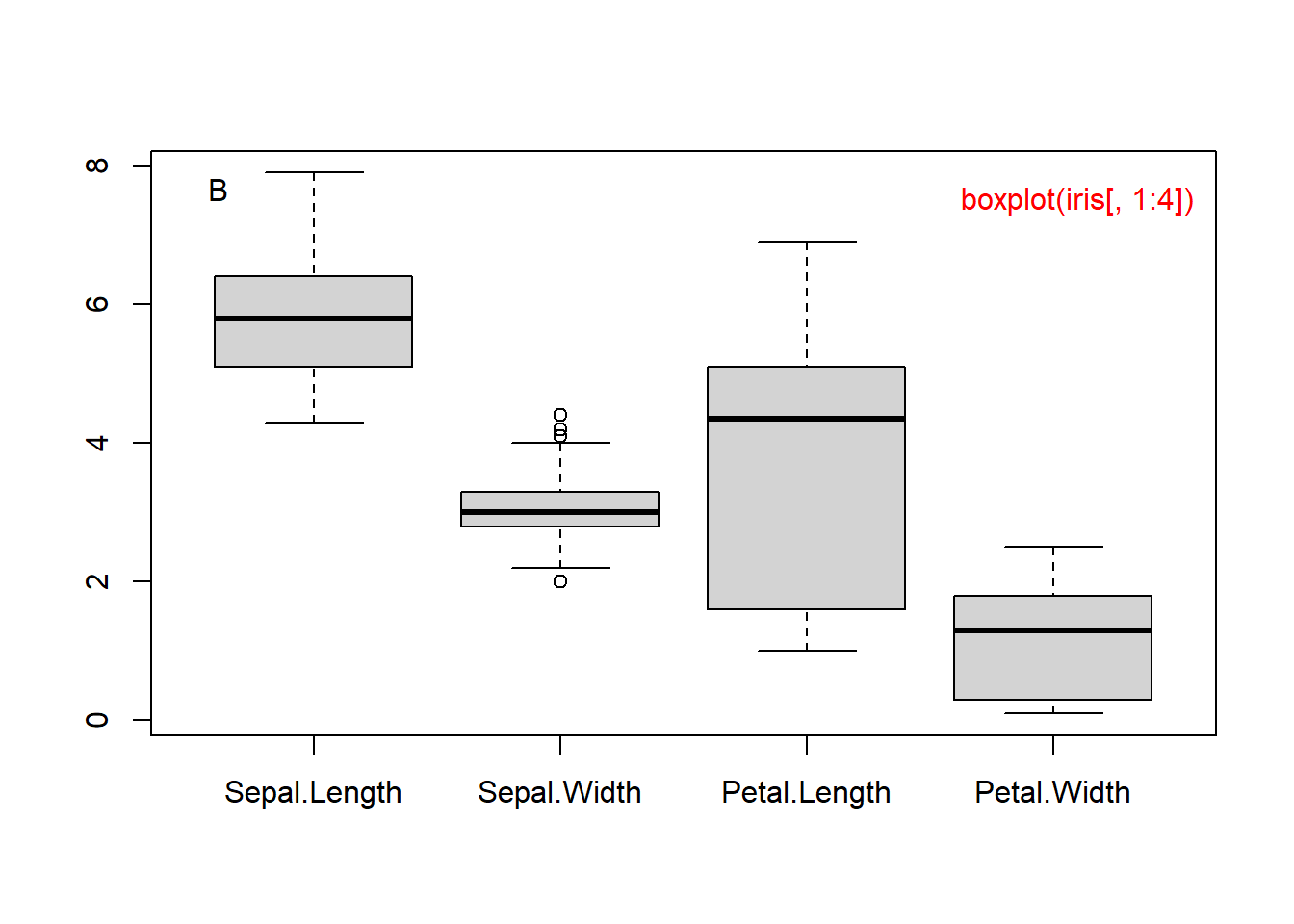Figure 1.3: Boxplot of petal length (A) and of all 4 columns (B).

In RStudio, you can copy a plot to the clipboard using the Export button on top of the plot area. Or you can click zoom, right click on the popup plot and select “Copy Image”. Then you can paste the plot into Word.

Exercise 1.4

1. Check the data structure of the built-in data set mtcars.
2. Get the boxplot of Mile Per Gallon (mpg) in the data set mtcars.

To quantify the variance, we can compute the standard deviation σ: \begin{align} σ=\sqrt{\frac{1}{N-1}[(x_{1}-\bar x)^2+(x_{2}-\bar x)^2+...+(x_{N}-\bar x)^2]} \end{align} where \begin{align} \bar x=\frac{1}{N}(x_{1}+x_{2}+...x_{N}) \end{align} If all the measurements are close to the mean (µ), then standard deviation should be small.

sd(PL)  # standard deviation
##  1.765298
sd(Sepal.Width) 
##  0.4358663

As we can see, these flowers have similar sepal width, but they differ widely in petal length. This is consistent with the boxplot above. Perhaps changes in petal length lead to better survival in different habitats.

With R, it is easy to generate graphs.

barplot(PL)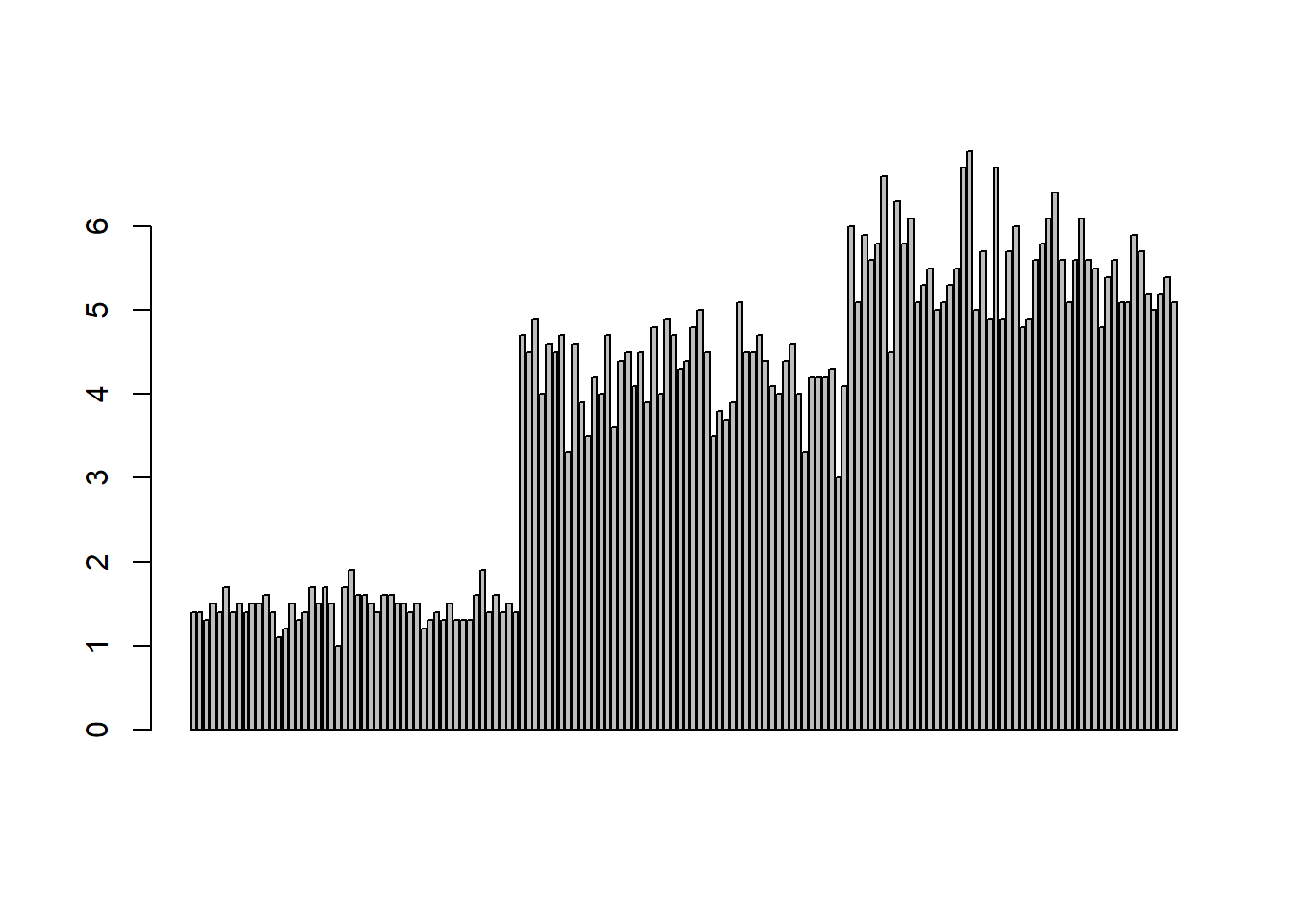Figure 1.4: Barplot of petal length.

This figure suggests that the first 50 flowers (Iris setosa) have much shorter petals than the other two species. The last 50 flowers (Iris virginica) have slightly longer petals than those of Iris versicolor.

plot(PL)  # Run scatter plot
hist(PL)  # histogram
lag.plot(PL)
qqnorm(PL)  # Q-Q plot for normal distribution
qqline(PL)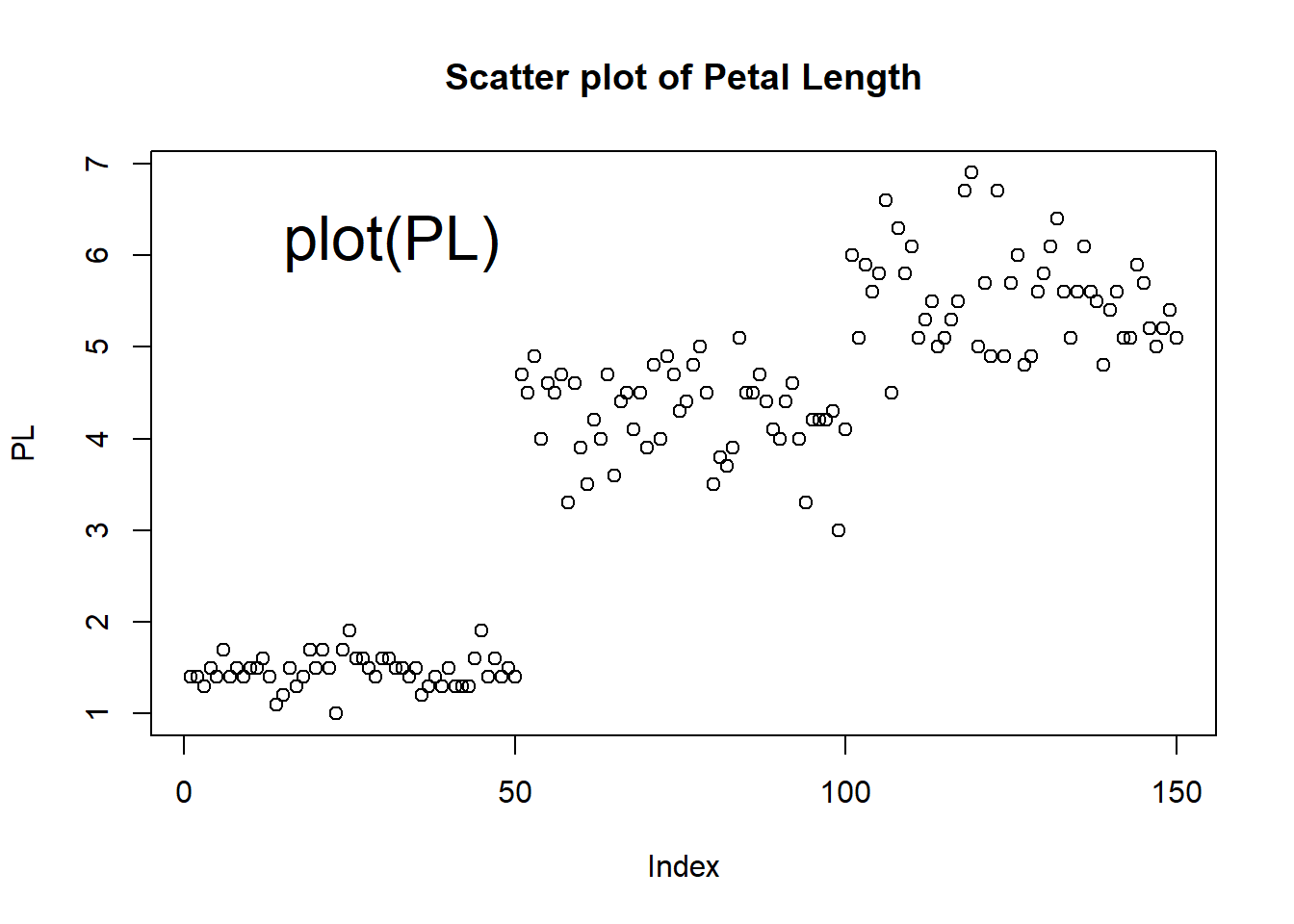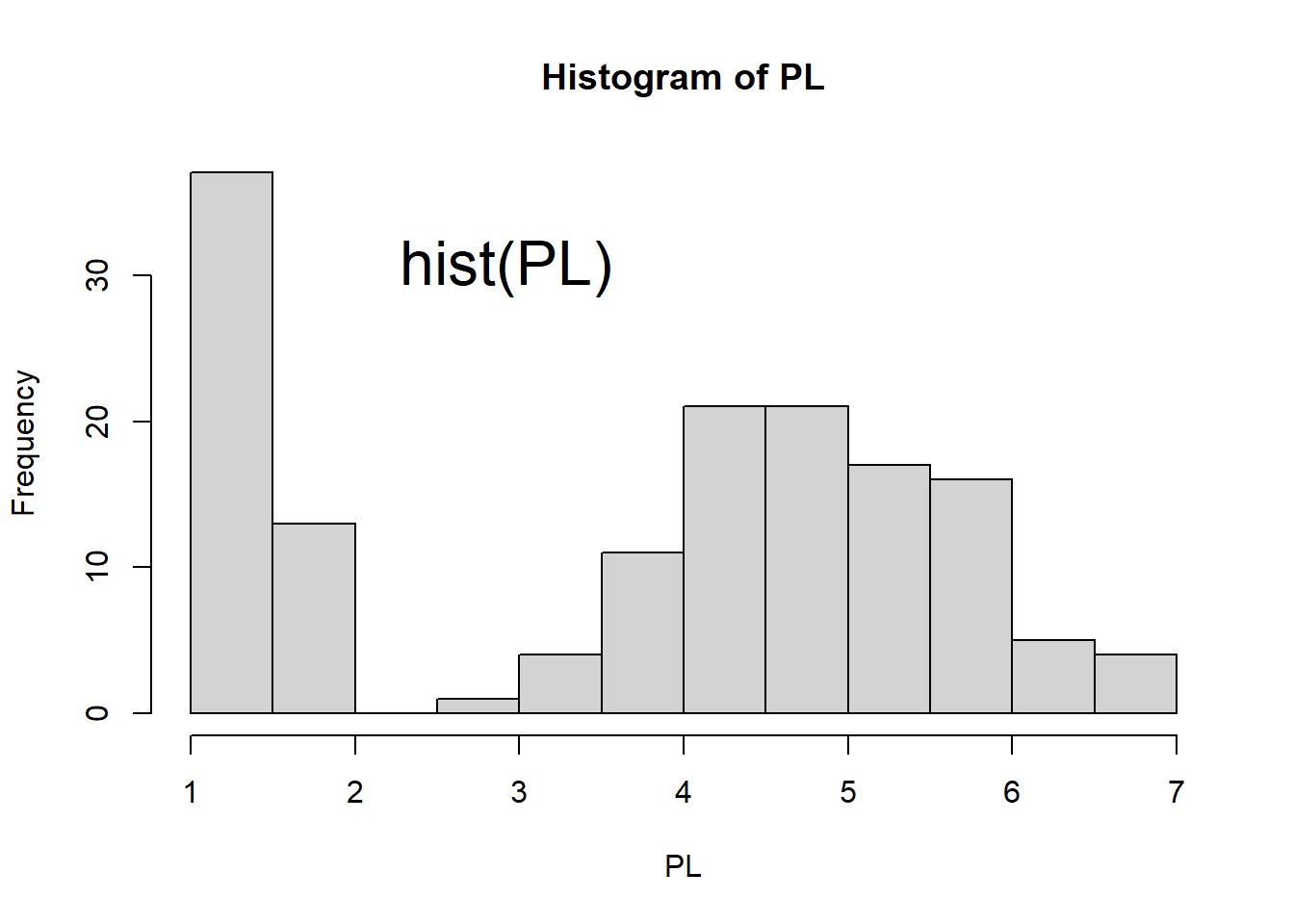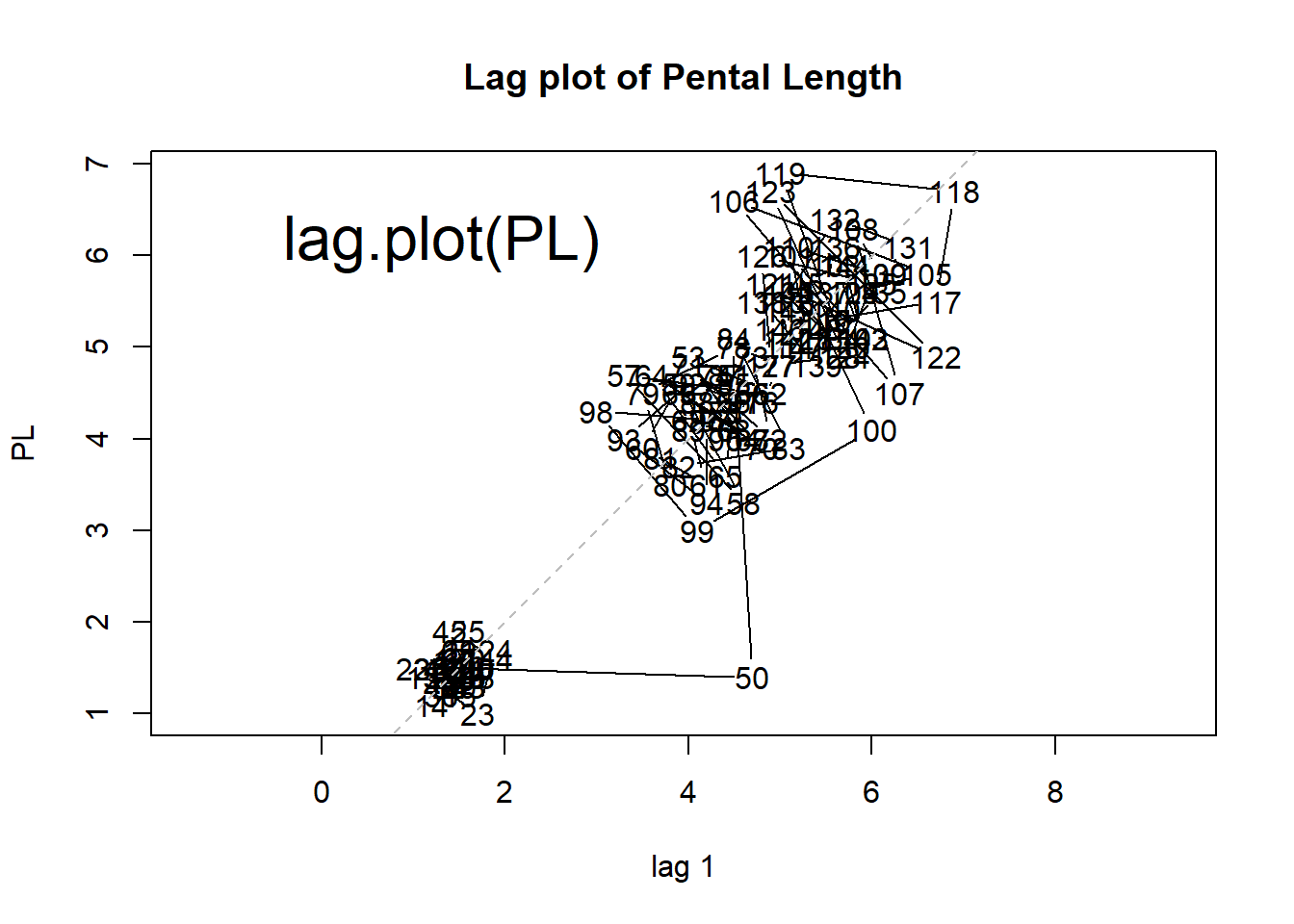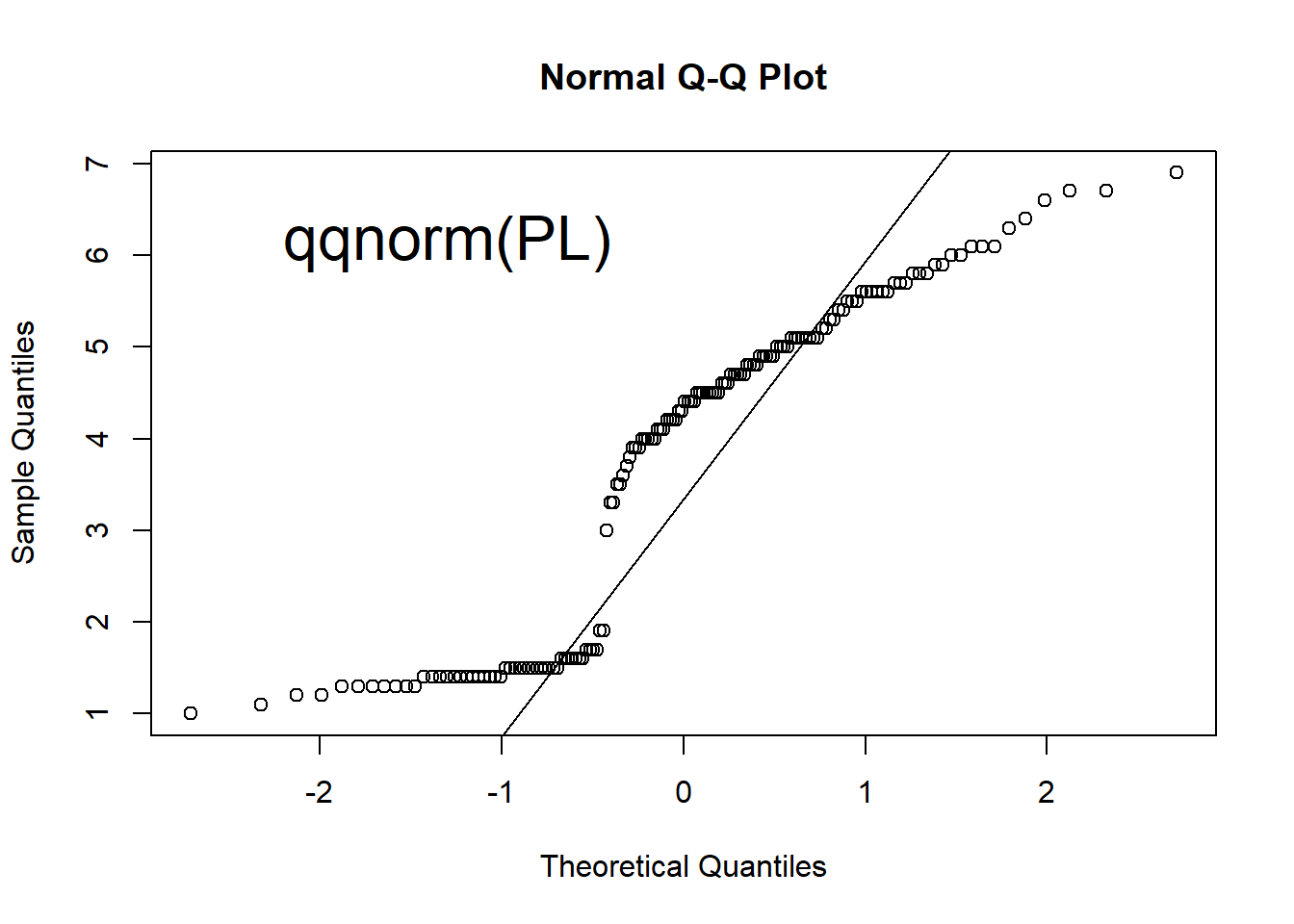Figure 1.5: Scatter plot, histogram, lag plot and normal Q-Q plot.

There are three different groups in the scatter plot. The petal length of one group is much smaller than the other two groups.

Histogram shows the distribution of data by plotting the frequency of data in bins. The histogram top right of Figure 1.5 shows that there are more flowers with Petal Length between 1 and 1.5. It also shows that the data does not show a bell-curved distribution.

The lag plot is a scatter plot against the same set of number with an offset of 1. Any structure in a lag plot indicates non-randomness in the order in which the data is presented. We can clearly see three clusters, indicating that values are centered around three levels sequentially.

Q-Q (quantile-quantile) plots can help check if data follows a Gaussian distribution, which is widely observed in many situations. Also referred to as normal distribution, it is the pre-requisite for many statistical methods. See Figure 1.6 for an example of normal distribution. Quantiles of the data is compared against those in a normal distribution. If the data points on a Q-Q plot form a straight line, the data has a normal distribution.

Exercise 1.5

1. Run x = rnorm(500) to generate 500 random numbers following the Standard Normal distribution
2. Generate scatter plot, histogram, lag plot, and Q-Q plot. Your plots should like those in Figure 1.6.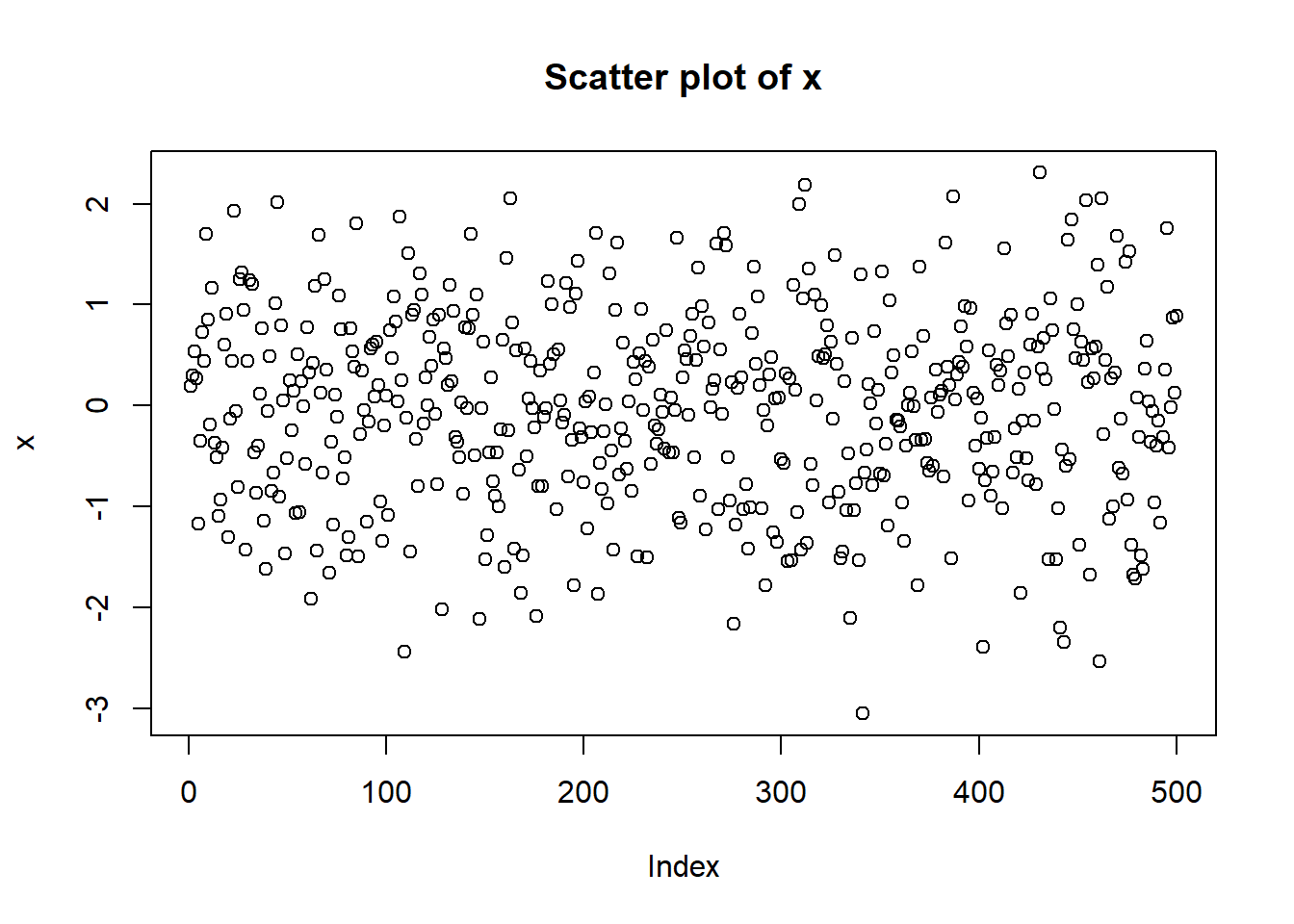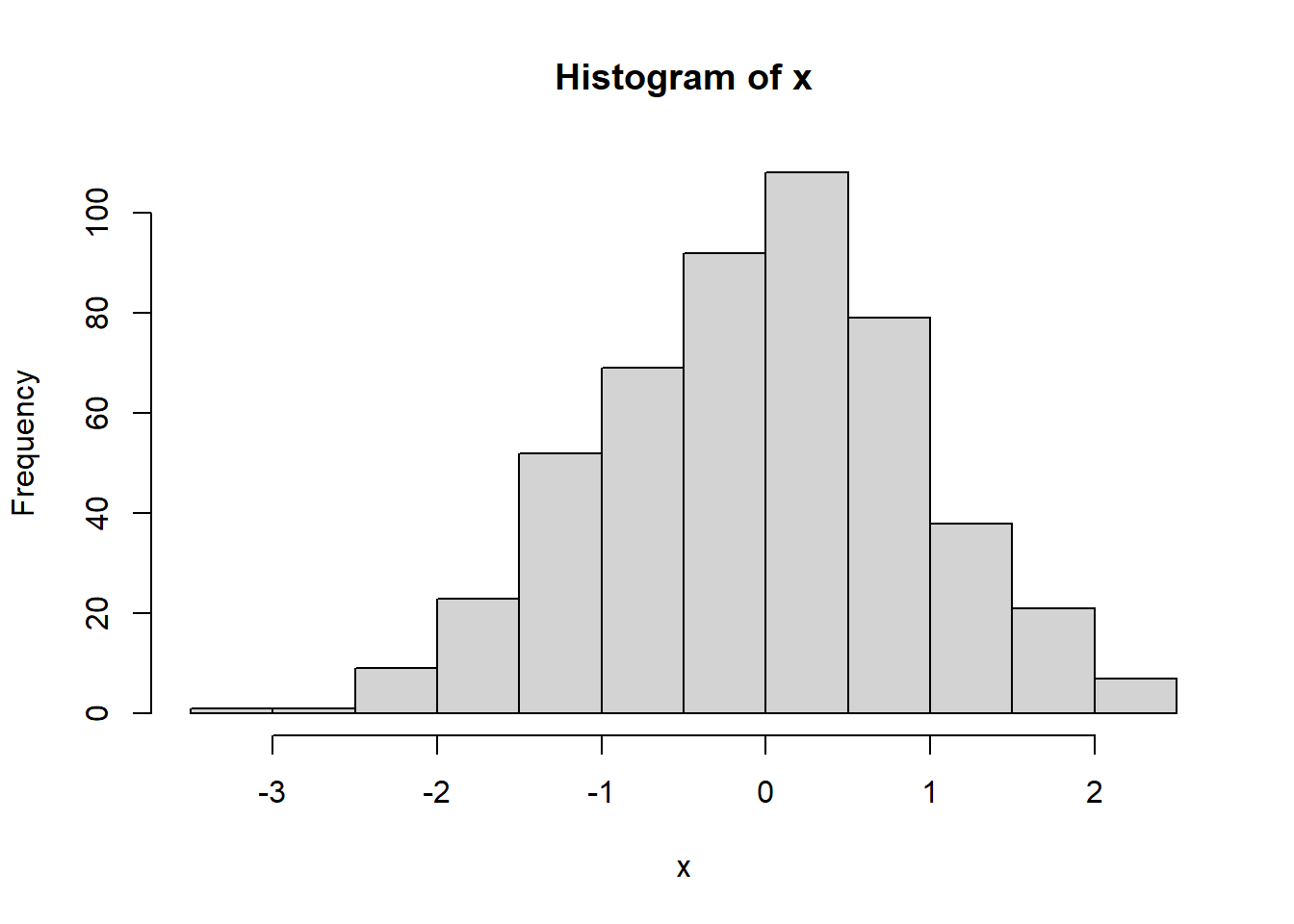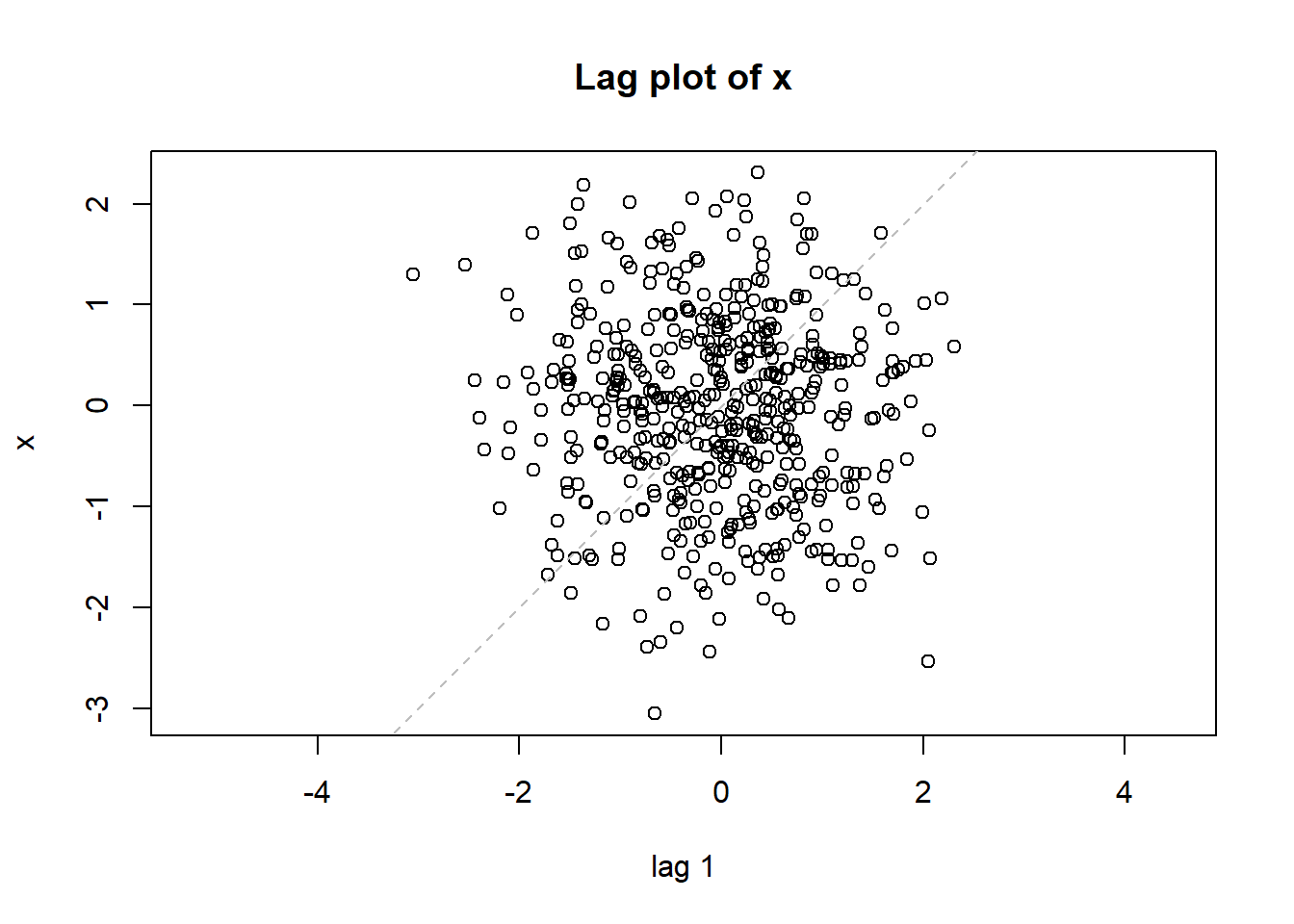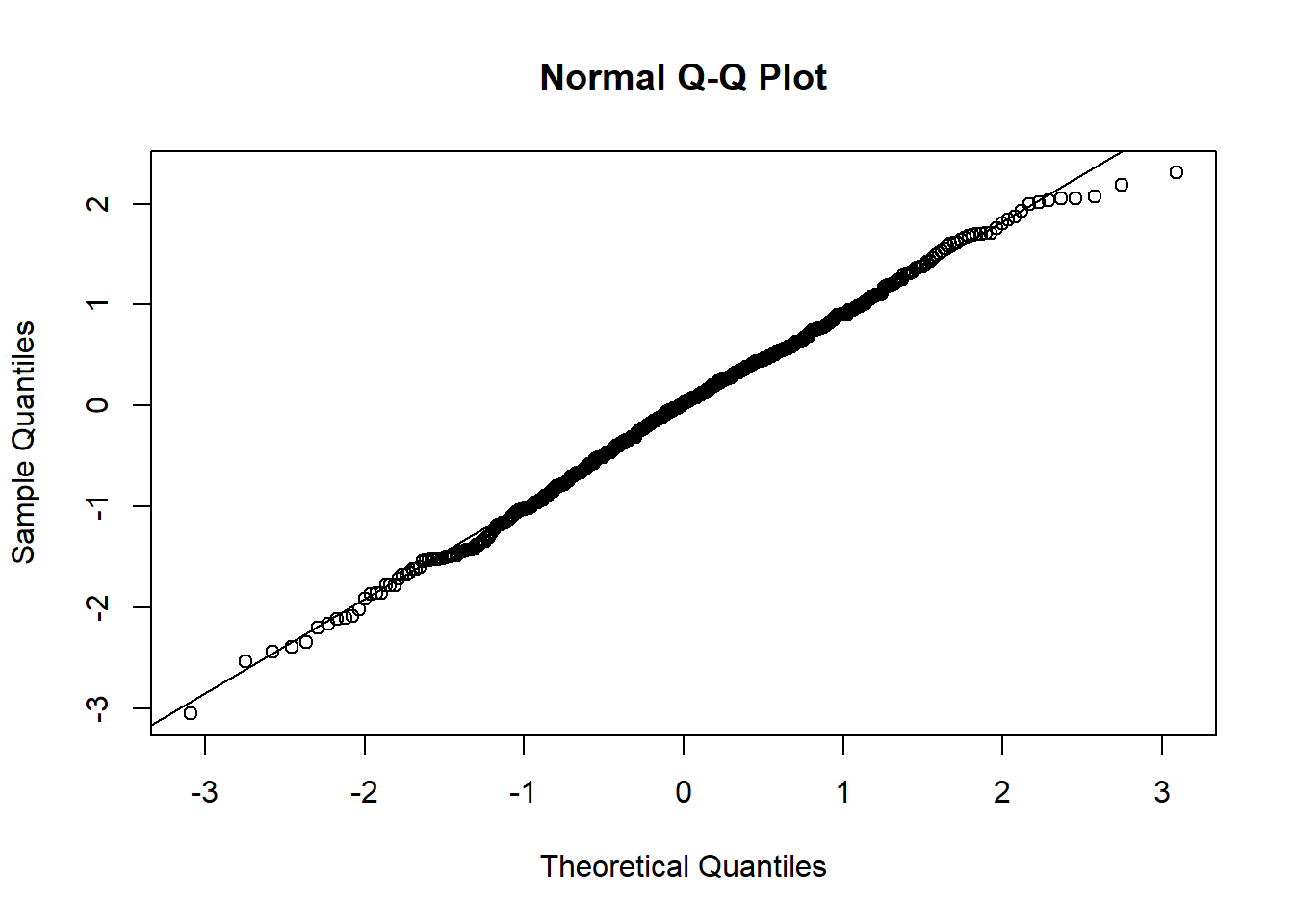Figure 1.6: Plots for randomly generated numbers following a normal distribution.

Exercise 1.6

Generate scatter plot, histogram, lag plot, and Q-Q plot for the Septal length in the iris dataset.

We can also do a one-sample t-test of mean:

t.test(PL, mu = 3.7)
##
##  One Sample t-test
##
## data:  PL
## t = 0.4024, df = 149, p-value = 0.688
## alternative hypothesis: true mean is not equal to 3.7
## 95 percent confidence interval:
##  3.473185 4.042815
## sample estimates:
## mean of x
##     3.758

In this case, our null hypothesis is that the true average of petal length for all iris flowers is 3.7. Since p-value is quite big, there is no evidence to reject this hypothesis. This function also tells us the 95% confidence interval on the mean. Based on our sample of 150 iris flowers, we are 95% confident that the true mean (if we were to meaure all iris flowers in the world) is between 5.71 and 5.98.

Exercise 1.7

Compute 95% confidence interval of sepal length.

We can perform hypothesis testing on whether a set of numbers are derived from normal distribution. When interpreting results from hypothesis testing, it is important to start by examining what the null hypothesis is. Here the null hypothesis is that the data is from a normal distribution.

shapiro.test(PL)
##
##  Shapiro-Wilk normality test
##
## data:  PL
## W = 0.87627, p-value = 7.412e-10

If petal length is normally distributed, there is only 7.412×10-10 chance of getting the observed test statistic of 0.87627. In other words, it is highly unlikely that petal length follows a normal distribution. We reject the normal distribution hypothesis, which could be corroborated by our plots above. In statistics, we rely on both charts and statistical models.

Exercise 1.8

1. Run Shapiro’s test on sepal width. Does it follow a normal distribution given the significant level is 0.05?
2. Generate histogram and Q-Q plot for sepal width. Do the plots show a Normal approximation?

## 1.4 Analyzing a categorical variable

In the iris dataset, the last column contains the species information. We call this a categorical variable. Bar and Pie charts are very effective in showing proportions. We can see that the three species are each represented with 50 observations.

counts <- table(Species)  # tabulate the frequencies
counts
## Species
##     setosa versicolor  virginica
##         50         50         50
pie(counts)  # See Figure 1.7A
barplot(counts)  # See Figure 1.7B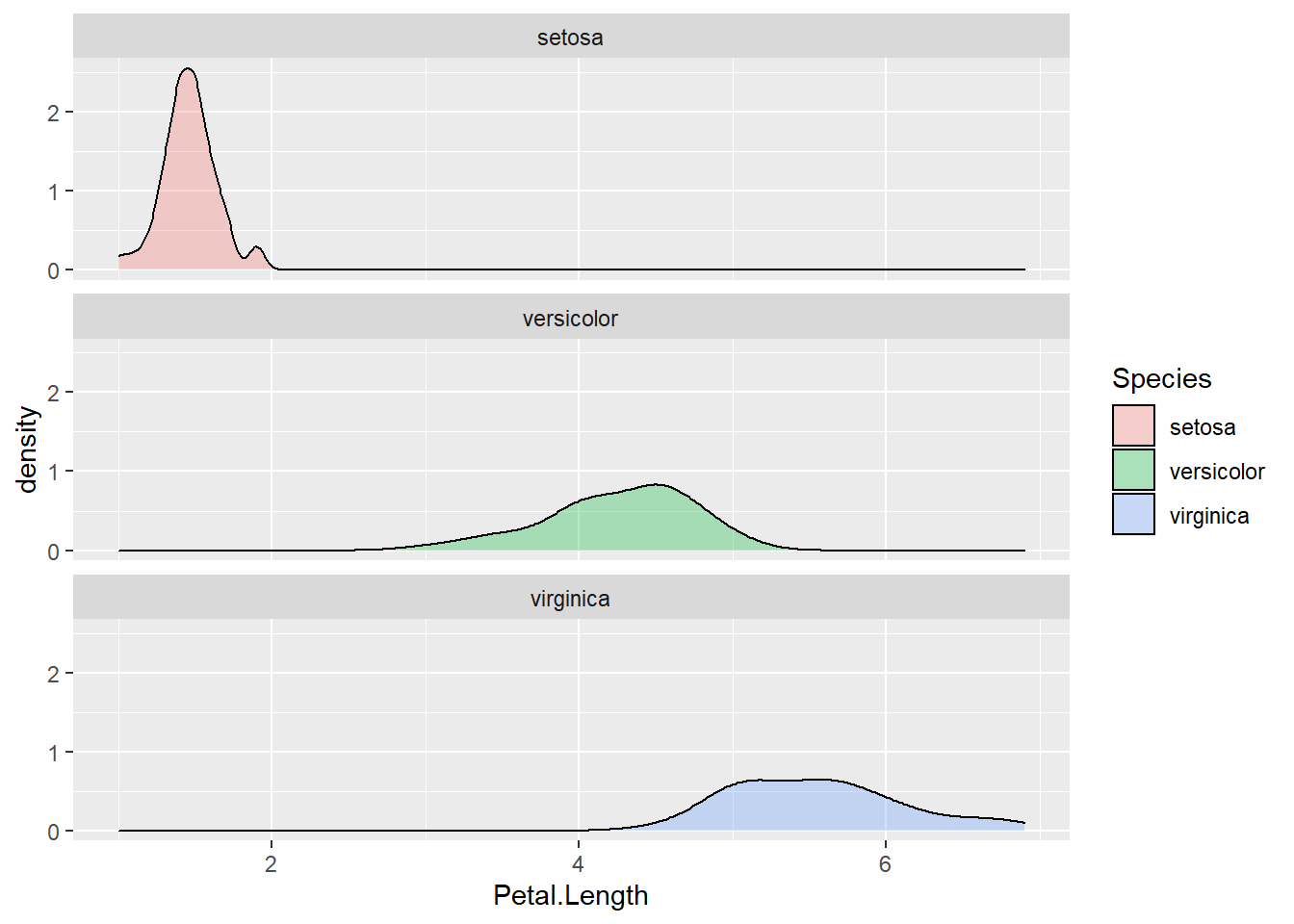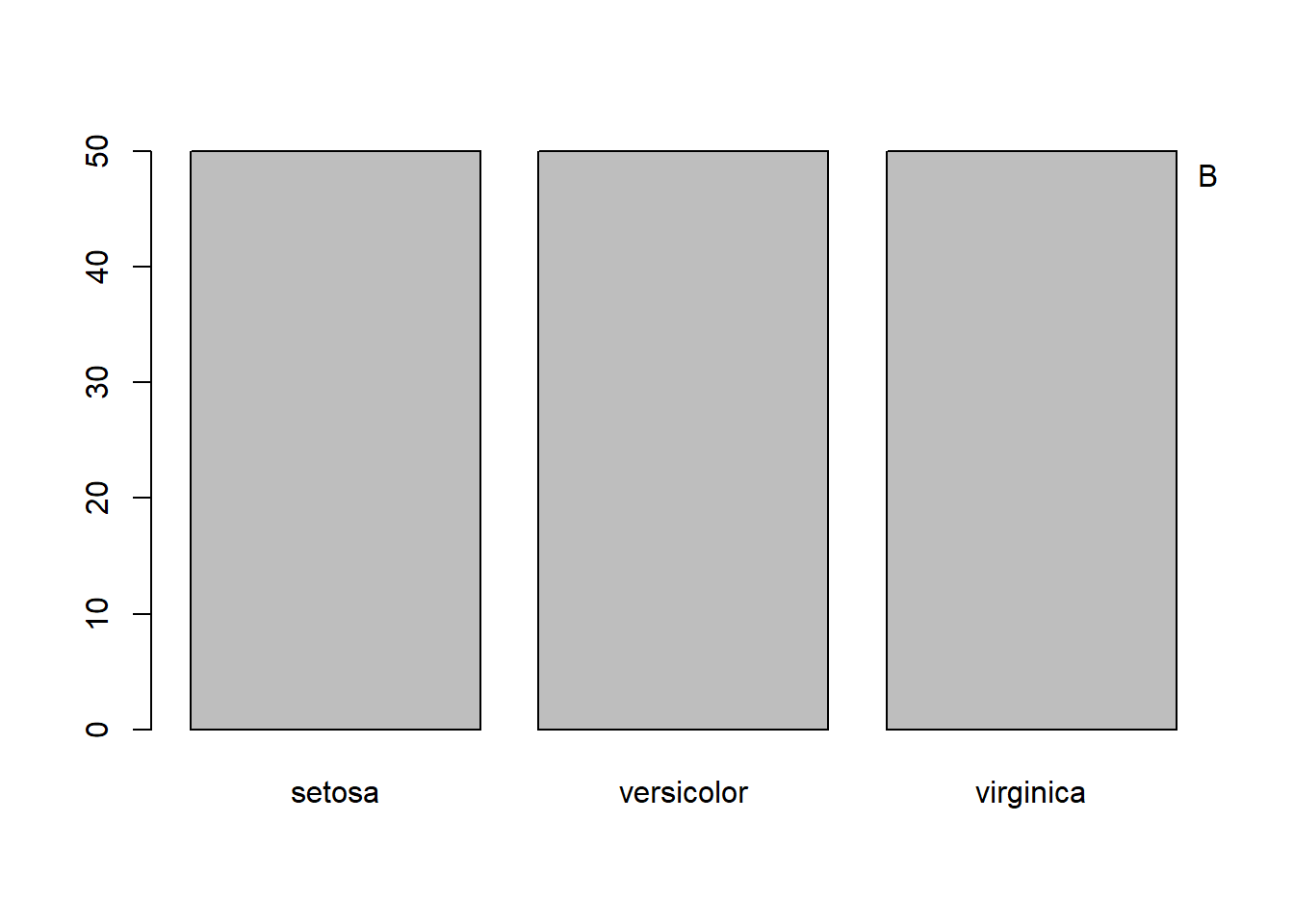Figure 1.7: Frequencies of categorical values visualized by Pie chart (A) and bar chart (B).

## 1.5 The relationship between two numerical variables

Scatter plot is very effective in visualizing the correlation between two columns of numbers.

PW <- iris$Petal.Width # just lazy plot(PW, PL) # scatterplot, refined version in Figure 1.9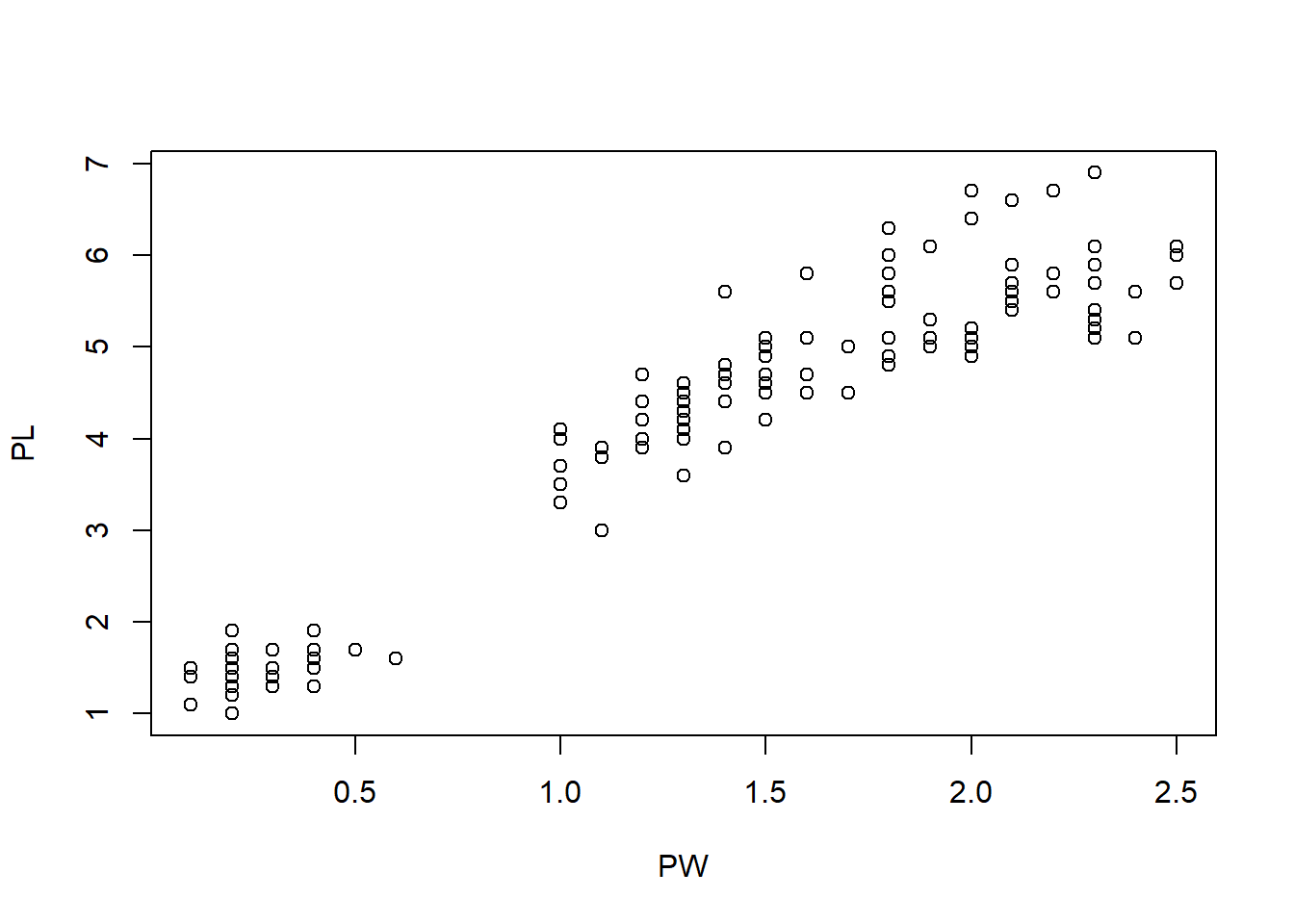Figure 1.8: Scatter plot of petal width and petal length. Figure 1.8 shows that there is a positive correlation between petal length and petal width. In other words, flowers with longer petals are often wider. So the petals are getting bigger substantially when both dimensions increase. Another unusual feature is that there seem to be two clusters of points. Do the points in the small cluster represent one particular species of Iris? We need to further investigate this. The following will produce a plot with the species information color-coded. The resultant Figure 1.9 clearly shows that indeed one particular species, I. setosa constitutes the smaller cluster in the low left. The other two species also show a difference in this plot, even though they are not easily separated. This is a very important insight into this dataset. SP <- as.factor(iris$Species)
plot(PW, PL, col = rainbow(3)[SP])  # change colors based on Species
legend("topleft", levels(SP), fill = rainbow(3))  # add legends on topleft.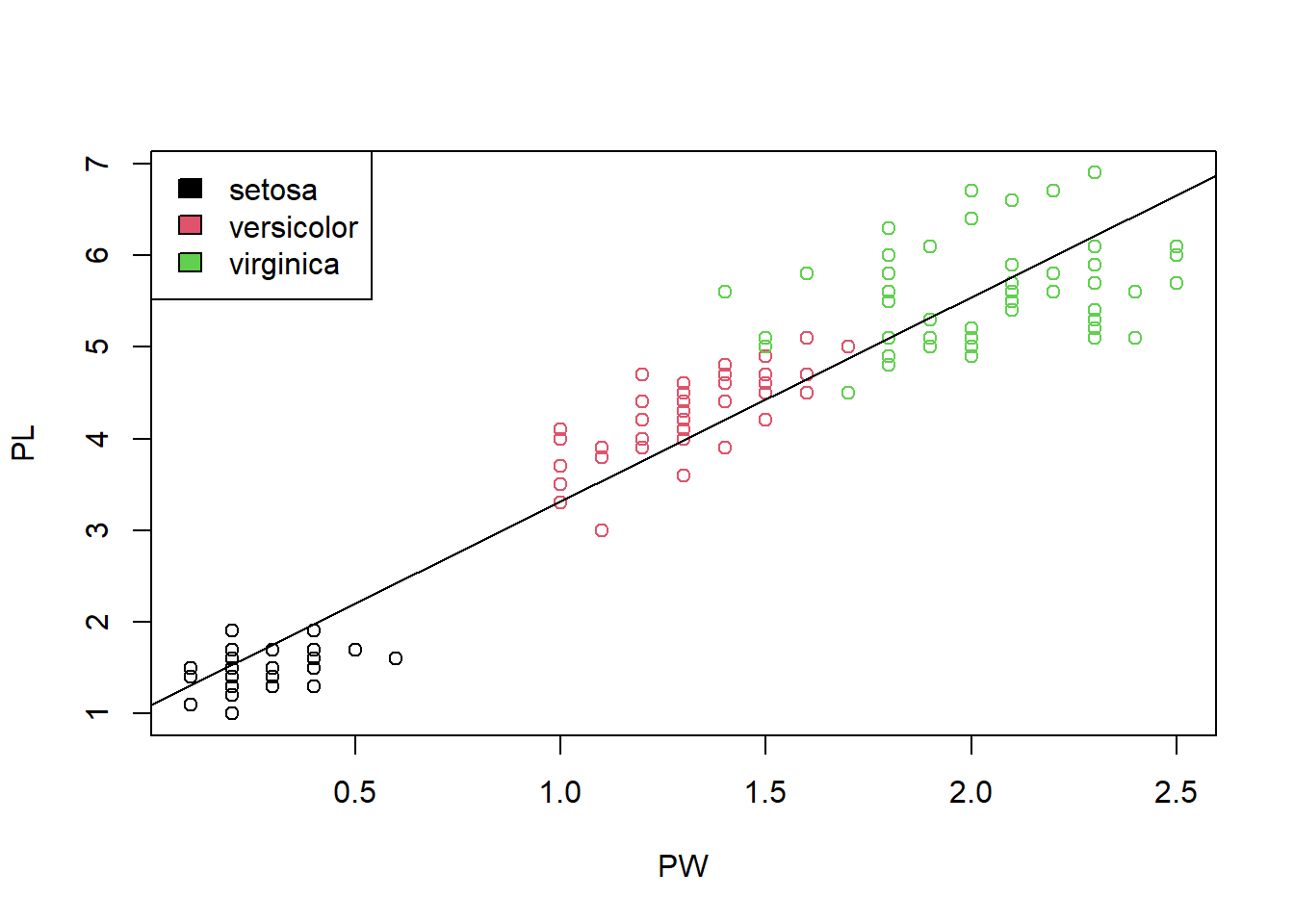Figure 1.9: Scatter plot shows the correlation of petal width and petal length.

We generate a vector of 3 colors using the rainbow() function. The 3 colors will correspond the 3 species. To change the color for a specific data point, we defined a new variable SP by converting the last column into a factor. The class(Species) returns the data structure of SP is a “factor.” The levels(Species) function shows all levels of Species. The function str(Species) shows that the Species is a factor with 3 levels which are internally coded as 1, 2, and 3. This enables us to change the colors. This kind of base plotting has been simplified by modern systems such as ggplot2, which we will discuss later. So you do not have to remember all of this.

str(SP)    # show the structure of Species.
##  Factor w/ 3 levels "setosa","versicolor",..: 1 1 1 1 1 1 1 1 1 1 ...

Perhaps due to adaption to the environment, change in petal length leads to better survival. With the smallest petals, Iris Setosa is found in Arctic regions. Iris versicolor is often found in the Eastern United States and Eastern Canada. Iris virginica “is common along the coastal plain from Florida to Georgia in the Southeastern United States–Wikipedia.” It appears the iris flowers in warmer places are much larger than those in colder ones. With R, it is very easy to generate lots of graphics. But we still have to do the thinking. It requires us to interpret the charts in the context.

Exercise 1.9

The mtcars data description can be found here: https://stat.ethz.ch/R-manual/R-patched/RHOME/library/datasets/html/mtcars.html.

Based on mtcars data set, plot a scatter plot which shows the correlation between Miles/(US) gallon and Displacement (cu.in.). In this data set, the type of cyl is numeric. You will need to use function newcyl -> as.factor(cyl) to transfer the type to factor. Then replace all mtcars\$cyl with newcyl.

1. Color the scatter plot by Number of cylinders;
2. Add legend to the top right.

We can quantitatively characterize the strength of the correlation using several types of correlation coefficients, such as Pearson’s correlation coefficient. It ranges from -1 to 1. Zero means no correlation.

cor(PW, PL) 
##  0.9628654

This means the petal width and petal length are strongly and positively correlated. we can add this informtion on the plot as text:

text(1.5, 1.5, paste("R=0.96"))
cor.test(PW, PL)
##
##  Pearson's product-moment correlation
##
## data:  PW and PL
## t = 43.387, df = 148, p-value < 2.2e-16
## alternative hypothesis: true correlation is not equal to 0
## 95 percent confidence interval:
##  0.9490525 0.9729853
## sample estimates:
##       cor
## 0.9628654

Through hypothesis testing, we reject the null hypothesis that the true correlation is zero (no correlation). That means the correlation is statistically significant. Note that Pearson’s correlation coefficient is not robust against outliers and other methods such as Spearman’s exists. See help info:

?cor  # show help info on cor ( )

We can also determine the equation that links petal length and petal width. This is so called regression analysis. We assume

$$Petal.Length = \alpha × Petal.Width + c + e$$,

where $$\alpha$$ is the slope parameter, $$c$$ is a constant, and $$e$$ is some random error. This linear model can be determined by a method that minimizes the least squared-error:

model <- lm(PL ~ PW)  # Linear Model (lm)
summary(model)      # shows the details
##
## Call:
## lm(formula = PL ~ PW)
##
## Residuals:
##      Min       1Q   Median       3Q      Max
## -1.33542 -0.30347 -0.02955  0.25776  1.39453
##
## Coefficients:
##             Estimate Std. Error t value Pr(>|t|)
## (Intercept)  1.08356    0.07297   14.85   <2e-16 ***
## PW           2.22994    0.05140   43.39   <2e-16 ***
## ---
## Signif. codes:  0 '***' 0.001 '**' 0.01 '*' 0.05 '.' 0.1 ' ' 1
##
## Residual standard error: 0.4782 on 148 degrees of freedom
## Multiple R-squared:  0.9271, Adjusted R-squared:  0.9266
## F-statistic:  1882 on 1 and 148 DF,  p-value: < 2.2e-16

Note that in the regression model, the tilde(~) links a response variable on the right with one or more independent variables on the right side. It is a shortcut for an operator. We are defining a statistical model where the PL is modeled as a function of PW. In addition to regression models, we also use formula in plots.

As we can see, we estimated that $$\alpha=2.22944$$ and $$c=1.08356$$. Both parameters are significantly different from zero as the p-values are <2×10-16 in both cases. In other words, we can reliably predict

$$Petal.Length = 2.22944 × Petal.Width + 1.08356$$.

This model can be put on the scatter plot as a line.

abline(model)  # add regression line to plot. Figure 1.9

And we can get the diagnostic plots for regression analysis:

plot(model)    # diagnostic plots for regression

Sometimes, we use this type of regression analysis to investigate whether variables are significantly associated.

Exercise 1.10

Investigate the relationship between sepal length and sepal width using scatter p lots, correlation coefficients, test of correlation, and linear regression. Again interpret all your results in PLAIN and proper English.

## 1.6 Testing the differences between two groups

Are boys taller than girls of the same age? Such situations are common. We have measurements of a random sample from a population. From the sample we want to know if the observed differences among the two groups reflect real differences in the population or due to random sampling error.

boxplot(Petal.Length ~ Species)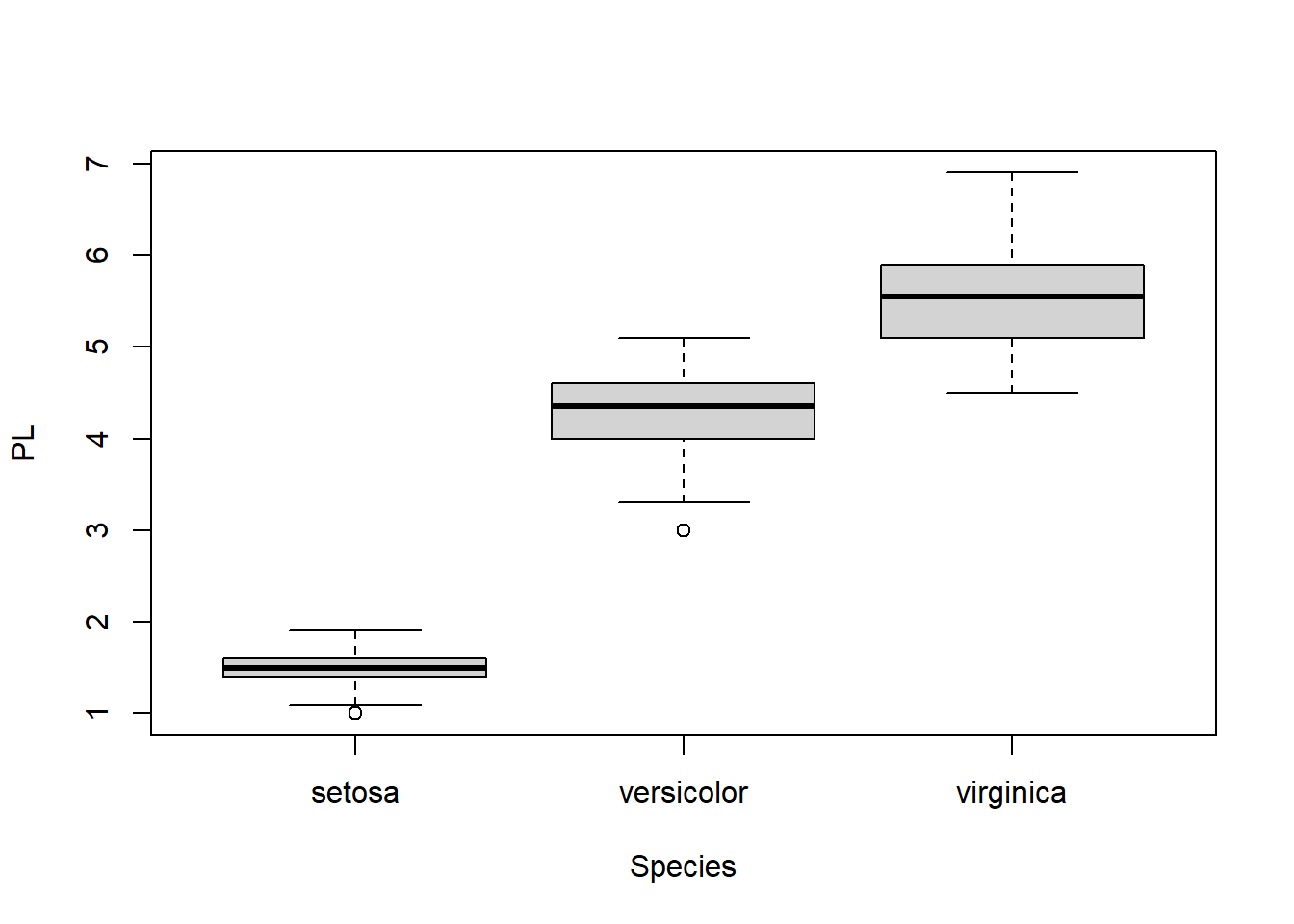Figure 1.10: Boxplot of petal length, grouped by species.

From the boxplot, it is obvious that I. Setosa has much shorter petals. But are there significant differences between I. versicolor and I. virginica? We only had a small sample of 50 flowers for each species. But we want to draw some conclusion about the two species in general. We could measure all the iris flowers across the world; Or we could use statistics to make inference. First we need to extract these data:

PL1 <- Petal.Length[51:100]  # extract Petal Length of iris versicolor
PL1  # a vector with 50 numbers
PL2 <- Petal.Length[101:150]  # extract Petal length of iris virginica
PL2  # y contain 50 measurements
boxplot(PL1, PL2)  # a boxplot of the two groups of values
t.test(PL1, PL2)
##
##  Welch Two Sample t-test
##
## data:  PL1 and PL2
## t = -12.604, df = 95.57, p-value < 2.2e-16
## alternative hypothesis: true difference in means is not equal to 0
## 95 percent confidence interval:
##  -1.49549 -1.08851
## sample estimates:
## mean of x mean of y
##     4.260     5.552

In this Student’s t-test, our null hypothesis is that the mean petal length is the same for I. versicolor and I. virginica. A small p-value of 2.2x10-16 indicates under this hypothesis, it is extremely unlikely to observe the difference of 1.292cm through random sampling. Hence we reject that hypothesis and conclude that the true mean is different. If we measure all I. versicolor and I. virginica flowers in the world and compute their true average petal lengths, it is very likely that the two averages will differ. On the other hand, if p-value is larger than a threshold, typically 0.05, we will accept the null hypothesis and conclude that real average petal length is the same.

We actually do not need to separate two set of numbers into two data objects in order to do t-test. We can do it right within the data frame. R can separate data points by another column.

df <- iris[51:150, ]  # Extract rows 51 to 150
t.test(Petal.Length ~ Species, data = df)  # t-test
boxplot(Petal.Length ~ Species, data = droplevels(df))  #removes empty levels in Species

Exercise 1.11

Use boxplot and t-test to investigate whether sepal width is different between I. versicolor and I. virginica. Interpret your results.

## 1.7 Testing the difference among multiple groups (ANOVA)

As indicated by Figure 1.11, sepal width has small variation across species. We want to know if the mean sepal width is the same across 3 species. This is done through Analysis of Variance (ANOVA).

boxplot(Sepal.Width ~ Species)  # Figure 1.11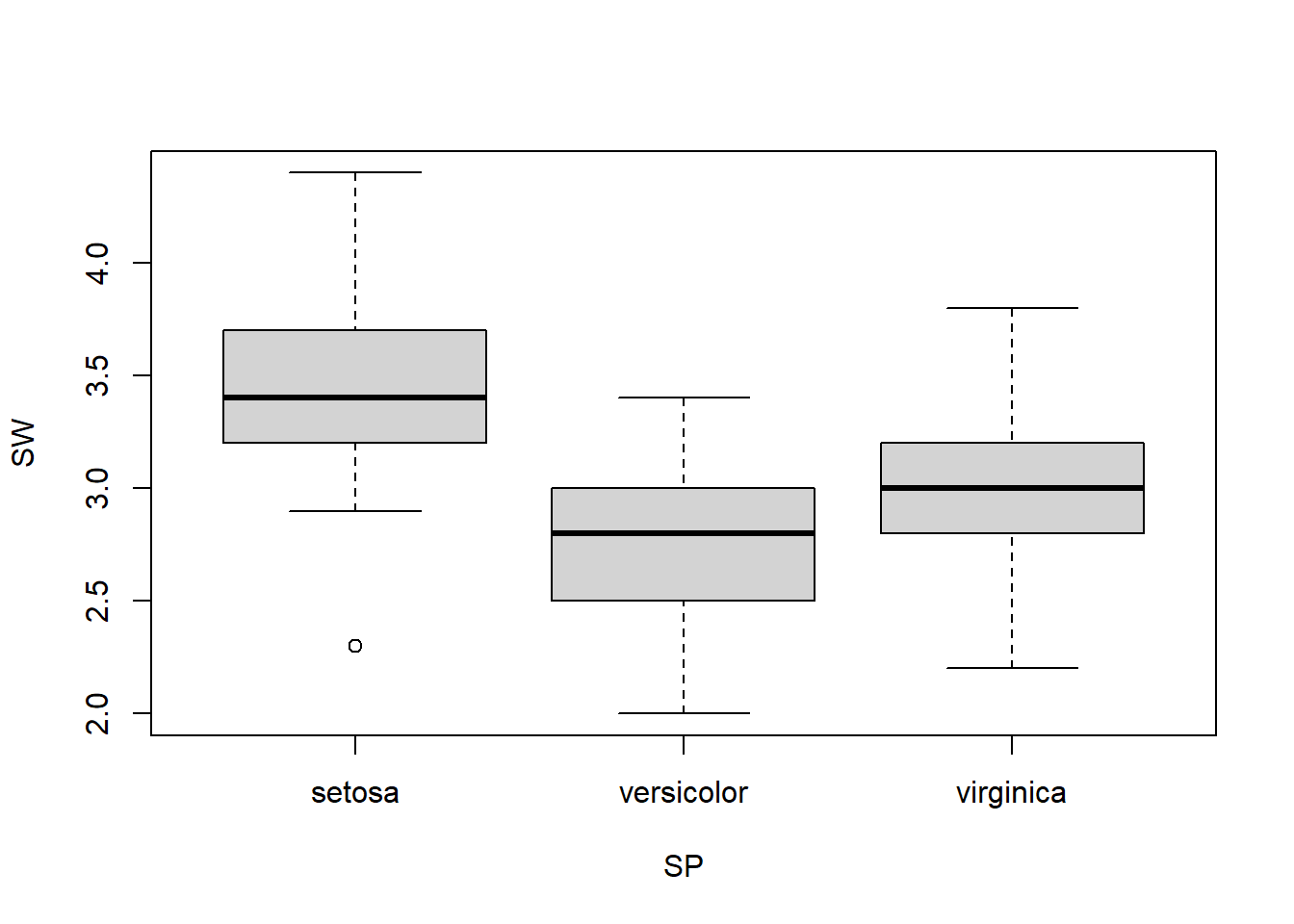Figure 1.11: Boxplot of sepal width across 3 species.

summary( aov(Sepal.Width ~ Species) )
##              Df Sum Sq Mean Sq F value Pr(>F)
## Species       2  11.35   5.672   49.16 <2e-16 ***
## Residuals   147  16.96   0.115
## ---
## Signif. codes:  0 '***' 0.001 '**' 0.01 '*' 0.05 '.' 0.1 ' ' 1

Since p-value is much smaller than 0.05, we reject the null hypothesis. The mean sepal width is not the same for 3 species. This is the only thing we can conclude from this, since this is how the hypothesis was set up. The boxplot in Figure 1.11 seems to indicate that I. Setosa has wider sepals.

Exercise 1.12

Use boxplot and ANOVA to investigate whether petal width is the same among three subspecies.

I hope this give you some idea about what it is like to using R to visualize and analyze data. It is interactive and graphical interface make it easy to manipulate data.From Tenor.com